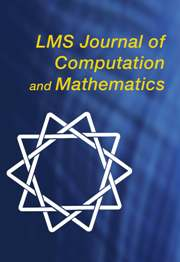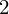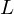Home
Hostname: page-component-cf9d5c678-xvx2z Total loading time: 0.347 Render date: 2021-08-03T12:09:06.024Z Has data issue: true Feature Flags: { "shouldUseShareProductTool": true, "shouldUseHypothesis": true, "isUnsiloEnabled": true, "metricsAbstractViews": false, "figures": true, "newCiteModal": false, "newCitedByModal": true, "newEcommerce": true, "newUsageEvents": true }LMS Journal of Computation and Mathematics

# A database of genus-2 curves over the rational numbers

Published online by Cambridge University Press:  26 August 2016

Corresponding

## Abstract

We describe the construction of a database of genus-$2$ curves of small discriminant that includes geometric and arithmetic invariants of each curve, its Jacobian, and the associated$L$ -function. This data has been incorporated into the$L$ -Functions and Modular Forms Database (LMFDB).

Type
Research Article
Information

## References

Baker, M. H., González-Jiménez, E., González, J. and Poonen, B., ‘Finiteness results for modular curves of genus at least 2’, Amer. J. Math. 127 (2005) 1325–1387.CrossRefGoogle Scholar
Birch, B. J. and Kuyk, W. (eds), Modular functions of one variable IV, Lecture Notes in Mathematics 476 (Springer, Berlin, 1975).Google Scholar
Booker, A. R., ‘Numerical tests of modularityJ. Ramanujan Math. Soc. 20 (2005) no. 4, 283–339.Google Scholar
Bosma, W., Cannon, J., Fieker, C. and Steel, A. (eds), Handbook of Magma functions, edition 2.16 (2010) 5017 pages.Google Scholar
Bosma, W., Cannon, J. and Playoust, C., ‘The Magma algebra system I: The user language’, J. Symbolic Comput. 24 (1997) 235–265.CrossRefGoogle Scholar
Brumer, A. and Kramer, K., ‘The conductor of an abelian variety’, Compositio Math. 92 (1994) 227–248.Google Scholar
Brumer, A. and Kramer, K., ‘Paramodular abelian varieties of odd conductor’, Trans. Amer. Math. Soc. 366 (2014) 2463–2516.CrossRefGoogle Scholar
Cardona, G., Nart, E. and Pujolas, J., ‘Curves of genus two over fields of even characteristic’, Math. Z. 250 (2005) 177–201.CrossRefGoogle Scholar
Cardona, G. and Quer, J., ‘Field of moduli and field of definition for curves of genus 2’, Computational aspects of algebraic curves, Lecture Notes Series on Computing 13 (World Scientific, Hackensack, NJ, 2005) 71–83.Google Scholar
Cremona, J. E., Algorithms for modular elliptic curves, 2nd edn (Cambridge University Press, Cambridge, 1997).Google Scholar
Cremona, J. E., ‘The elliptic curve database for conductors to 130000’, Algorithmic number theory: 7th international symposium, ANTS-VII, Lecture Notes in Computer Science 4076 (eds F. Hess, S. Pauli and M. E. Pohst; Springer, Berlin, 2006) 11–29.Google Scholar
Dokchitser, T., ‘Computing special values of motivic L-functions’, Exp. Math. 13 (2004) 137–149.CrossRefGoogle Scholar
Dupont, R., ‘Moyenne arithmético-géométrique, suites de Borchardt et applications’, PhD Thesis, Laboratoire d’Informatique de l’École Polytechnique, 2006, available at http://www.lix.polytechnique.fr/Labo/Regis.Dupont/these_soutenance.pdf.Google Scholar
Farmer, D., Koutsoliotas, S. and Lemurell, S., ‘Varieties via their L-functions’, Preprint, 2015, arXiv:1502.00850v1.Google Scholar
Fité, F., Kedlaya, K. S., Rotger, V. and Sutherland, A. V., ‘Sato–Tate distributions and Galois endomorphism modules in genus 2’, Compositio Math. 148 (2012) 1390–1442.CrossRefGoogle Scholar
González, J., Guàrdia, J. and Rotger, V., ‘Abelian surfaces of GL$_2$-type as Jacobians of curves’, Acta Arith. 116 (2005) 263–287.CrossRefGoogle Scholar
González, J. and Rotger, V., ‘Equations of Shimura curves of genus two’, Int. Math. Res. Not. IMRN 2004 (2004) no. 14, 661–674.Google Scholar
González-Jiménez, E. and González, J., ‘Modular curves of genus 2’, Math. Comp. 72 (2002) 397–418.CrossRefGoogle Scholar
González-Jiménez, E., González, J. and Guàrdia, J., ‘Computations on modular Jacobian surfaces’, Algorithmic number theory: 5th international symposium, ANTS-V, Lecture Notes in Computer Science 2369 (eds C. Fieker and D. R. Kohel; Springer, Berlin, 2002) 189–197.Google Scholar
Harvey, D. and Sutherland, A. V., ‘Computing Hasse-Witt matrices of hyperelliptic curves in average polynomial time’, Algorithmic number theory (ANTS XI), LMS J. Comput. Math. 17 (2014) 257–273.CrossRefGoogle Scholar
Harvey, D. and Sutherland, A. V., ‘Computing Hasse-Witt matrices of hyperelliptic curves in average polynomial time II’, Frobenius distributions: Lang–Trotter and Sato–Tate conjectures, Contemporary Mathematics 663 (eds D. Kohel and I. E. Shparlinski; American Mathematical Society, Providence, RI, 2016), 127–148, to appear.Google Scholar
Holt, D., ‘The meataxe as a tool in computational group theory’, The atlas of finite groups: ten years on (Birmingham, 1995), London Mathematical Society Lecture Note Series 249 (Cambridge University Press, Cambridge, 1998) 74–81.Google Scholar
Igusa, J.-I., ‘Arithmetic variety of moduli for genus two’, Ann. of Math. (2) 72 (1960) 612–649.CrossRefGoogle Scholar
Johansson, F., ‘Arb: a C library for ball arithmetic’, ACM Commun. Comput. Algebra 47 (2013) no. 4, 166–169.Google Scholar
Kedlaya, K. S. and Sutherland, A. V., ‘Computing L-series of hyperelliptic curves’, Algorithmic number theory: 8th international symposium, ANTS-VIII, Lecture Notes in Computer Science 5011 (eds A. J. van der Poorten and A. Stein; Springer, Berlin, 2008) 312–326.Google Scholar
Lenstra, A. K., Lenstra, H. W. Jr. and Lovász, L., ‘Factoring polynomials with rational coefficients’, Math. Ann. 261 (1982) 513–534.CrossRefGoogle Scholar
Liu, Q., ‘Conducteur et discriminant minimal de courbes de genre 2’, Compositio Math. 94 (1994) 51–79.Google Scholar
Liu, Q., ‘Modèles minimaux des courbes de genre deux’, J. reine angew. Math. 453 (1994) 137–164.Google Scholar
Liu, Q., ‘Modèles entiers des courbes hyperelliptiques sur un corps valuation discrète’, Trans. Amer. Math. Society 348 (1996) 4577–4610.CrossRefGoogle Scholar
Lockhart, P., ‘On the discriminant of a hyperelliptic curve’, Trans. Amer. Math. Soc. 342 (1994) 729–752.CrossRefGoogle Scholar
Merriman, J. R. and Smart, N. P., ‘Curves of genus 2 with good reduction away from 2 with a rational Weierstrass point’, Math. Proc. Cambridge Philos. Soc. 114 (1993) 203–214.CrossRefGoogle Scholar
Mestre, J.-F., ‘Construction de courbes de genre 2 à partir de leurs modules’, Effective methods in algebraic geometry, Progress in Mathematics 94 (Birkhäuser, Boston, MA, 1991) 313–334.Google Scholar
The PARI-Group, PARI/GP version 2.7.0 Bordeaux, 2014, available at http://pari.math.u-bordeaux.fr/.Google Scholar
Poonen, B., ‘Computational aspects of curves of genus at least 2’, Algorithmic number theory (ANTS II), Lecture Notes in Computer Science 1122 (ed. H. Cohen; Springer, Berlin, 1996) 283–306.Google Scholar
Smart, N. P., ‘S-unit equations, binary forms, and curves of genus 2’, Proc. Lond. Math. Soc. (3) 75 (1997) 271–307.CrossRefGoogle Scholar
Soundararajan, K., ‘Strong multiplicity one for the Selberg class’, Canad. Math. Bull. 47 (2004) 468–474.CrossRefGoogle Scholar
Sutherland, A. V., smalljac, version 4.1.3, available at http://math.mit.edu/∼drew.Google Scholar
Stein, W. and Watkins, M., ‘A database of elliptic curves—first report’, Algorithmic number theory: 5th international symposium, ANTS-V, Lecture Notes in Computer Science 2369 (eds C. Fieker and D. R. Kohel; Springer, Berlin, 2002) 267–275.Google Scholar
van Wamelen, P. B., ‘Computing with the analytic Jacobian of a genus 2 curve’, Discovering mathematics with Magma, Algorithms and Computation in Mathematics 19 (Springer, Berlin, 2006) 117–135.Google ScholarYou have Access
9
Cited by

# Send article to Kindle

Note you can select to send to either the @free.kindle.com or @kindle.com variations. ‘@free.kindle.com’ emails are free but can only be sent to your device when it is connected to wi-fi. ‘@kindle.com’ emails can be delivered even when you are not connected to wi-fi, but note that service fees apply.

Find out more about the Kindle Personal Document Service.

A database of genus-2 curves over the rational numbers
Available formats
×

# Send article to Dropbox

To send this article to your Dropbox account, please select one or more formats and confirm that you agree to abide by our usage policies. If this is the first time you use this feature, you will be asked to authorise Cambridge Core to connect with your <service> account. Find out more about sending content to Dropbox.

A database of genus-2 curves over the rational numbers
Available formats
×

# Send article to Google Drive

To send this article to your Google Drive account, please select one or more formats and confirm that you agree to abide by our usage policies. If this is the first time you use this feature, you will be asked to authorise Cambridge Core to connect with your <service> account. Find out more about sending content to Google Drive.

A database of genus-2 curves over the rational numbers
Available formats
×
×